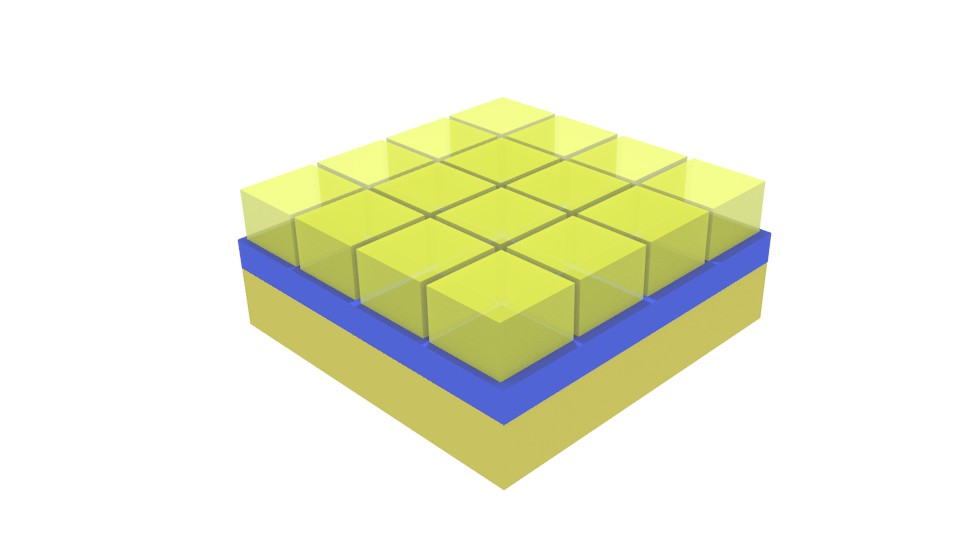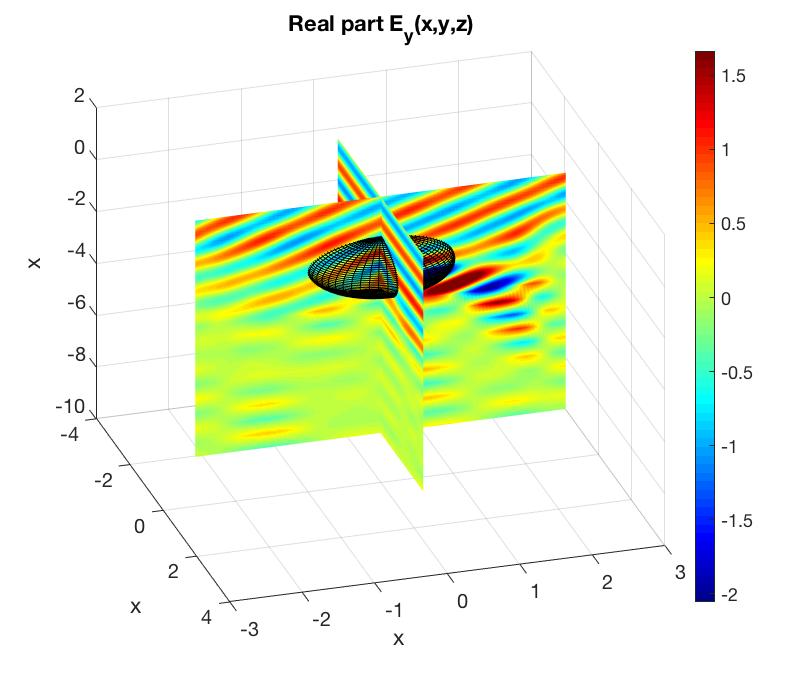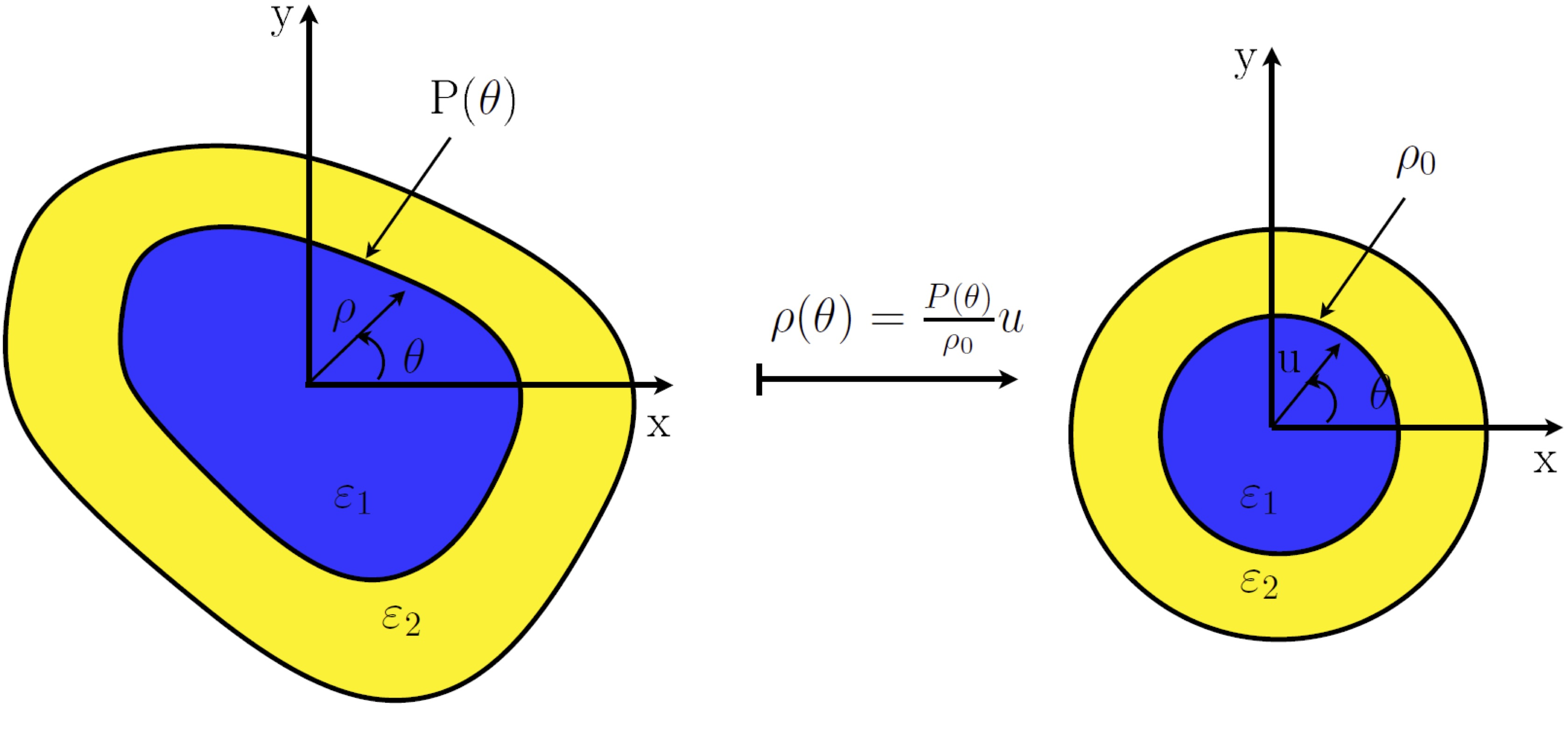### Periodic Fourier Modal Method (FMM)The Fourier modal method (FMM) introduced by Knop (1978) in the case of dielectric gratings, consists of approximating the eigenfunctions of the operator of diffraction by a partial Fourier sum. Moharam and Gaylord (1981) generalized this method to analyze diffraction by general planar gratings (or volume gratings) bounded by two different media and introduced the terminology: rigorous coupled wave analysis/method (RWCA or RWCM). Later on, Li (1998) adopted the terminology FMM, since the method is essentially a modal method that involved expansions of the electromagnetic field components and the permittivity function into Fourier series. The use of the name FMM is justified for at least two reasons:

• it means that the RCWA is not different from the method proposed by Knop
• It enters under a general terminology to be compared classical modal method (Botten et al., 1981a, 1981b), which is called in some cases "Modal Method Modal Expansion"

The case of metallic gratings, and especially the TM polarization case, challenged the com- munity until 1996, when Granet and Guizal and P. Lalanne and G. M. Morris6 (1996) simultaneously reformulated the FMM for isotropic gratings under transverse magnetic (TM) polarization by suggesting calculation rules allowing a fast convergence of the series of the partial Fourier sums. The mathematical justification of their findings was provided after by Li  (1996) under the form of three Fourier factorization rules.

### Polynomial Modal Method  for periodic and non periodic  strucutres (PMM/APMM)In the very recent years, the new improvements made on the modal methods based on polynomial decompositions (Legendre, Chebychev, Gegenbauer) of the fields, position them as serious competitors to the FMM even when it is equipped with the ASR concept.   Globally, two variants of the PMM have been suggested:

• First formulation of PMM

In the first formulation, a partition of the structure in the periodicity direction is defined. On each subinterval of the partition, corresponding to an homogeneous medium, the restriction of the eigenvectors is approximated by orthogonal polynomials (Gegenbauer ones for instance). These approximations are then introduced in Maxwell’s equations and an undersized matrix relation is obtained. The eigenvalue equation is deduced by adding to this undersized matrix relation the boundary conditions satisfied by the electromagnetic field components.

• Second formulation of PMM

However the connection of the boundary conditions to the undersized matrix is very technical and this process can be quite tedious. That is why we have introduced a second formulation which is conceptually synthetic and easier to implement. It consists in departing from an orthogonal set of polynomials and defining a set of modified polynomials basis that naturally and rigorously describe the transversal boundary conditions. One of the strengths of this version of the PMM, is that it allowed for an easy generalization to crossed lamellar gratings which would have been tedious with the former version.

Papers:

### PMM implementation in matched coordinatesPaper:

### 3D Aperiodic Fourier Modal Method (AFMM)  for dielectric problemThe aperiodic Fourier modal method (AFMM) is a hybrid method that combines a solver for periodic systems with perfectly matched layers (PMLs). The key challenge of implementing PMLs involves describing both the periodic incident plane wave (the input field) and the aperiodic scattered field (the output field) of the isolated device within the same formalism. To address this challenge, we introduce a hybrid method that combines a Fourier basis, Maxwell’s equations in complex coordinates, and the Stratton and Chu formulation.

### C-Method

The curvilinear coordinates method was applied to study the scattering of plane waves by a grating. The main point of this method is the choice of a coordinate system such that the profile of the surface coincides with a surface of coordinates. Furthermore, in the case of a translation curvilinear coordinate system, Maxwell's equations lead to an eigenvalue equation. Numerically, the eigenvalue equation is solved by using the method of moments with periodic or pseudoperiodic functions as expansion and test functions. The convergence of the method is closely related to the choice of the expansion functions that have to fit the boundary conditions of the problem. Hence, for grating problems, the choice of periodic functions is a natural one.